# 9.16. Chapter Assessment - List Methods¶

Which of these is a correct reference diagram following the execution of the following code?

lst = ['mercury', 'venus', 'earth', 'mars', 'jupiter', 'saturn', 'uranus', 'neptune', 'pluto']
lst.remove('pluto')
first_three = lst[:3]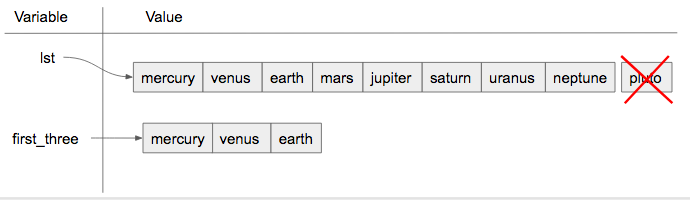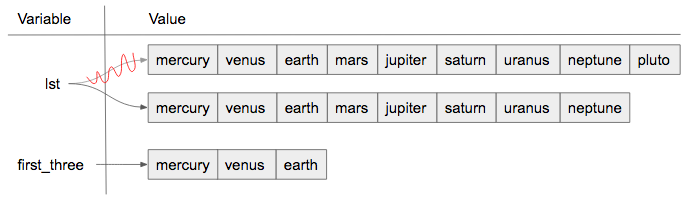• I.
• Yes, when we are using the remove method, we are just editing the existing list, not making a new copy.
• II.
• When we use the remove method, we just edit the existing list. We do not make a new copy that does not include the removed object.
• Neither is the correct reference diagram.
• One of the diagrams is correct - look again at what is happening to lst.

Which method would you use to figure out the position of an item in a list?

• .pop()
• pop removes and returns items (default is to remove and return the last item in the list)
• .insert()
• insert will add an item at whatever position is specified.
• .count()
• count returns the number of times something occurs in a list
• .index()
• Yes, index will return the position of the first occurance of an item.

Which method is best to use when adding an item to the end of a list?

• .insert()
• While you can use insert, it is not the best method to use because you need to specify that you want to stick the new item at the end.
• .pop()
• pop removes an item from a list
• .append()
• Yes, though you can use insert to do the same thing, you don't need to provide the position.
• .remove()
• remove gets rid of the first occurance of any item that it is told. It does not add an item.

Write code to add ‘horseback riding’ to the third position (i.e., right before volleyball) in the list sports.

Write code to take ‘London’ out of the list trav_dest.

Write code to add ‘Guadalajara’ to the end of the list trav_dest using a list method.

## 9.16.1. Chapter Assessment - Aliases and References¶

What will be the value of a after the following code has executed?

a = ["holiday", "celebrate!"]
quiet = a
quiet.append("company")


The value of a will be

Could aliasing cause potential confusion in this problem?

b = ['q', 'u', 'i']
z = b
b = 'i'
z.remove('i')
print(z)

• yes
• Yes, b and z reference the same list and changes are made using both aliases.
• no
• Can you figure out what the value of b is only by looking at the lines that mention b?

Could aliasing cause potential confusion in this problem?

sent = "Holidays can be a fun time when you have good company!"
phrase = sent
phrase = phrase + " Holidays can also be fun on your own!"

• yes
• Since a string is immutable, aliasing won't be as confusing. Beware of using something like item = item + new_item with mutable objects though because it creates a new object. However, when we use += then that doesn't happen.
• no
• Since a string is immutable, aliasing won't be as confusing. Beware of using something like item = item + new_item with mutable objects though because it creates a new object. However, when we use += then that doesn't happen.

Which of these is a correct reference diagram following the execution of the following code?

x = ["dogs", "cats", "birds", "reptiles"]
y = x
x += ['fish', 'horses']
y = y + ['sheep']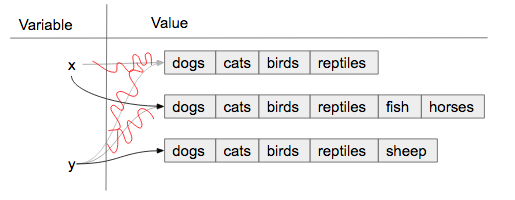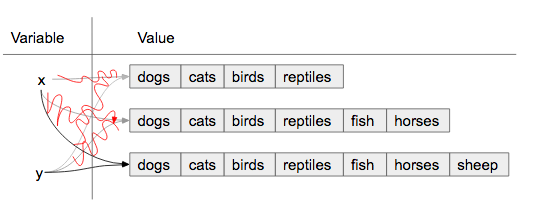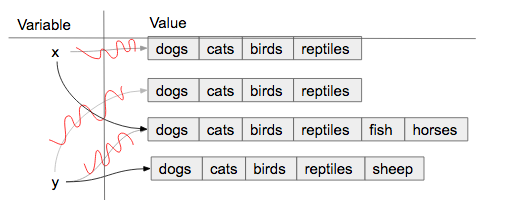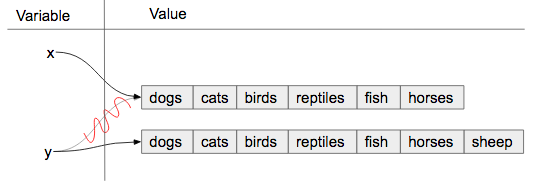• I.
• When an object is concatenated with another using +=, it extends the original object. If this is done in the longer form (item = item + object) then it makes a copy.
• II.
• When an object is concatenated with another using +=, it extends the original object. If this is done in the longer form (item = item + object) then it makes a copy.
• III.
• When an object is concatenated with another using +=, it extends the original object. If this is done in the longer form (item = item + object) then it makes a copy.
• IV.
• Yes, the behavior of obj = obj + object_two is different than obj += object_two when obj is a list. The first version makes a new object entirely and reassigns to obj. The second version changes the original object so that the contents of object_two are added to the end of the first.

## 9.16.2. Chapter Assessment - Split and Join¶

Which of these is a correct reference diagram following the execution of the following code?

sent = "The mall has excellent sales right now."
wrds = sent.split()
wrds = 'store'
new_sent = " ".join(wrds)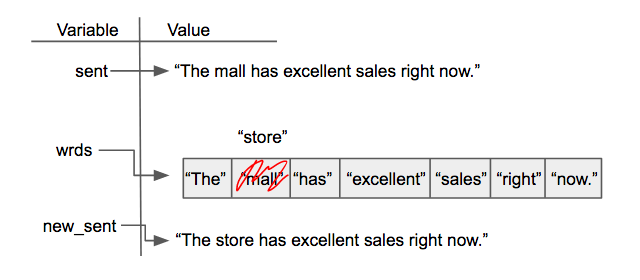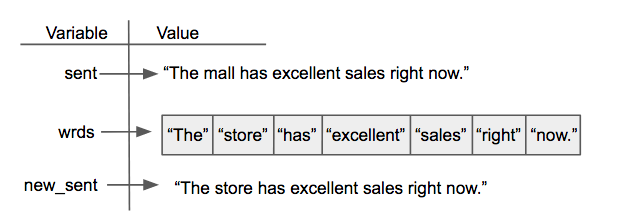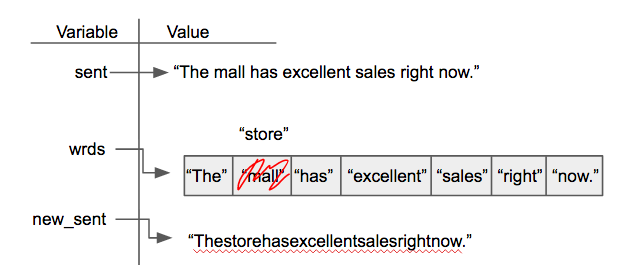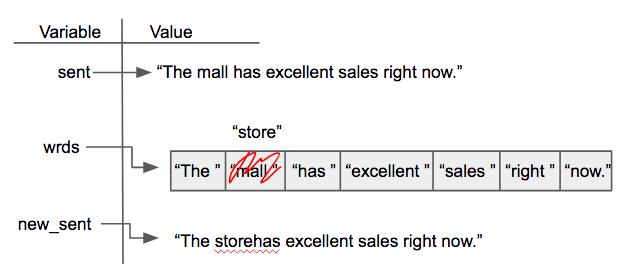• I.
• Yes, when we make our own diagrams we want to keep the old information because sometimes other variables depend on them. It can get cluttered though if there is a lot of information.
• II.
• Not quite, we want to keep track of old information because sometimes other variables depend on them.
• III.
• Look again at what is happening when join is executed.
• IV.
• What happens to the spaces in a string when it is split by whitespace?

Write code to find the position of the string “Tony” in the list awards and save that information in the variable pos.

## 9.16.3. Chapter Assessment - For Loop Mechanics¶

Which of these is the accumulator variable?

byzo = 'hello world!'
c = 0
for x in byzo:
z = x + "!"
print(z)
c = c + 1

• byzo
• This is the variable with our string, but it does not accumulate anything.
• x
• This is the iterator variable. It changes each time but does not accumulate.
• z
• This is a variable inside the for loop. It changes each time but does not accumulate or retain the old expressions that were assigned to it.
• c
• Yes, this is the accumulator variable. By the end of the program, it will have a full count of how many items are in byzo.

Which of these is the sequence?

cawdra = ['candy', 'daisy', 'pear', 'peach', 'gem', 'crown']
t = 0
for elem in cawdra:
t = t + len(elem)

• cawdra
• Yes, this is the sequence that we iterate over.
• elem
• This is the iterator variable. It changes each time but is not the whole sequence itself.
• t
• This is the accumulator variable. By the end of the program, it will have a full count of how many items are in cawdra.

Which of these is the iterator (loop) variable?

lst = [5, 10, 3, 8, 94, 2, 4, 9]
num = 0
for item in lst:
num += item

• item
• Yes, this is the iterator variable. It changes each time but is not the whole sequence itself.
• lst
• This is the sequence that we iterate over.
• num
• This is the accumulator variable. By the end of the program, it will have the total value of the integers that are in lst.

What is the iterator (loop) variable in the following?

rest = ["sleep", 'dormir', 'dormire', "slaap", 'sen', 'yuxu', 'yanam']
let = ''
for phrase in rest:
let += phrase


The iterator variable is

Currently there is a string called str1. Write code to create a list called chars which should contain the characters from str1. Each character in str1 should be its own element in the list chars.

## 9.16.4. Chapter Assessment - Accumulator Pattern¶

Given that we want to accumulate the total sum of a list of numbers, which of the following accumulator patterns would be appropriate?

nums = [4, 5, 2, 93, 3, 5]
s = 0
for n in nums:
s = s + 1

nums = [4, 5, 2, 93, 3, 5]
s = 0
for n in nums:
s = n + n

nums = [4, 5, 2, 93, 3, 5]
s = 0
for n in nums:
s = s + n

• I.
• This pattern will only count how many items are in the list, not provide the total accumulated value.
• II.
• This would reset the value of s each time the for loop iterated, and so by the end s would be assigned the value of the last item in the list plus the last item in the list.
• III.
• Yes, this will solve the problem.
• none of the above would be appropriate for the problem.
• One of the patterns above is a correct way to solve the problem.

Given that we want to accumulate the total number of strings in the list, which of the following accumulator patterns would be appropriate?

lst = ['plan', 'answer', 5, 9.29, 'order, items', ]
s = 0
for n in lst:
s = s + n

lst = ['plan', 'answer', 5, 9.29, 'order, items', ]
for item in lst:
s = 0
if type(item) == type("string"):
s = s + 1

lst = ['plan', 'answer', 5, 9.29, 'order, items', ]
s = ""
for n in lst:
s = s + n

lst = ['plan', 'answer', 5, 9.29, 'order, items', ]
s = 0
for item in lst:
if type(item) == type("string"):
s = s + 1

• 1.
• How does this solution know that the element of lst is a string and that s should be updated?
• 2.
• What happens to s each time the for loop iterates?
• 3.
• Reread the prompt again, what do we want to accumulate?
• 4.
• Yes, this will solve the problem.
• none of the above would be appropriate for the problem.
• One of the patterns above is a correct way to solve the problem.

Which of these are good names for an accumulator variable? Select as many as apply.

• sum
• No, though sum might be clear, it is also the name of a commonly used function in Python, and so there can be issues if sum is used as an accumulator variable.
• x
• No, x is not a clear enough name to be used for an accumulator variable.
• total
• Yes, total is a good name for accumulating numbers.
• accum
• Yes, accum is a good name. It's both short and easy to remember.
• none of the above
• At least one of the answers above is a good name for an accumulator variable.

Which of these are good names for an iterator (loop) variable? Select as many as apply.

• item
• Yes, item can be a good name to use as an iterator variable.
• y
• No, y is not likely to be a clear name for the iterator variable.
• elem
• Yes, elem can be a good name to use as an iterator variable, especially when iterating over lists.
• char
• Yes, char can be a good name to use when iterating over a string, because the iterator variable would be assigned a character each time.
• none of the above
• At least one of the answers above is a good name for an iterator variable.

Which of these are good names for a sequence variable? Select as many as apply.

• num_lst
• Yes, num_lst is good for a sequence variable if the value is actually a list of numbers.
• p
• No, p is not likely to be a clear name for the iterator variable.
• sentence
• Yes, this is good to use if the for loop is iterating through a string.
• names
• Yes, names is good, assuming that the for loop is iterating through actual names and not something unrelated to names.
• none of the above
• At least one of the answers above is a good name for a sequence variable

Given the following scenario, what are good names for the accumulator variable, iterator variable, and sequence variable? You are writing code that uses a list of sentences and accumulates the total number of sentences that have the word ‘happy’ in them.

• accumulator variable: x | iterator variable: s | sequence variable: lst
• Though lst is an acceptable name, x and s are not informative names for accumulator and iterator variables.
• accumulator variable: total | iterator variable: s | sequence variable: lst
• Though total is great and lst is an acceptable name, s is a little bit cryptic as a variable name referring to a sentence.
• accumulator variable: x | iterator variable: sentences | sequence variable: sentence_lst
• Though sentence_lst is a good name, the iterator variable should be singular rather than plural, and x is not an informative name for the accumulator variable.
• accumulator variable: total | iterator variable: sentence |sequence variable: sentence_lst
• Yes, this combination of variable names is the clearest.
• none of the above
• One of the options above has good names for the scenario.

For each character in the string saved in ael, append that character to a list that should be saved in a variable app.

For each string in wrds, add ‘ed’ to the end of the word (to make the word past tense). Save these past tense words to a list called past_wrds.

Write code to create a list of word lengths for the words in original_str using the accumulation pattern and assign the answer to a variable num_words_list. (You should use the len function).

Create an empty string and assign it to the variable lett. Then using range, write code such that when your code is run, lett has 7 b’s ("bbbbbbb").

## 9.16.5. Chapter Assessment - Problem Solving¶

Below are a set of scores that students have received in the past semester. Write code to determine how many are 90 or above and assign that result to the value a_scores.

Write code that uses the string stored in org and creates an acronym which is assigned to the variable acro. Only the first letter of each word should be used, each letter in the acronym should be a capital letter, and there should be nothing to separate the letters of the acronym. Words that should not be included in the acronym are stored in the list stopwords. For example, if org was assigned the string “hello to world” then the resulting acronym should be “HW”.

Write code that uses the string stored in sent and creates an acronym which is assigned to the variable acro. The first two letters of each word should be used, each letter in the acronym should be a capital letter, and each element of the acronym should be separated by a “. ” (dot and space). Words that should not be included in the acronym are stored in the list stopwords. For example, if sent was assigned the string “height and ewok wonder” then the resulting acronym should be “HE. EW. WO”.

A palindrome is a phrase that, if reversed, would read the exact same. Write code that checks if p_phrase is a palindrome by reversing it and then checking if the reversed version is equal to the original. Assign the reversed version of p_phrase to the variable r_phrase so that we can check your work.

Provided is a list of data about a store’s inventory where each item in the list represents the name of an item, how much is in stock, and how much it costs. Print out each item in the list with the same formatting, using the .format method (not string concatenation). For example, the first print statment should read The store has 12 shoes, each for 29.99 USD.